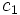# Column orthogonality theorem

This article gives the statement, and possibly proof, of a basic fact in linear representation theory.
View a complete list of basic facts in linear representation theory OR View all facts related to linear representation theory
This article describes an orthogonality theorem. View a list of orthogonality theorems

## Statement

Let$G$ be a finite group, and$k$ be a splitting field for$G$.

Then, consider the character table of$G$: this is a matrix whose rows are indexed by the irreducible linear representations of$G$ over$k$, and whose columns are indicated by the conjugacy classes of$G$, and where the entry in row$\rho$ and column$c$ is the trace of$\rho(g)$ where$g \in c$.

Then, the columns of this table are orthogonal. More explicitly, for any conjugacy classes$c_1$ and$c_2$, pick$g_1 \in c_1, g_2 \in c_2$. We get:$\sum_\chi \chi(g_1)\chi(g_2^{-1}) = 0$

where$\chi$ varies over the characters of irreducible linear representations of$G$.

Further, for a single conjugacy class$c$ and$g \in c$:$\sum_\chi \chi(g)\chi(g^{-1}) = \frac{|G|}{|c|}$

Note that any sufficiently large field (i.e., any field that contains all the$m^{th}$ roots of unity where$m$ is the exponent of$G$) is a splitting field.

## Proof

We use the row orthogonality theorem for the sufficiently large field case. By the row orthogonality theorem, we know that the rows of the character table are pairwise orthogonal with respect to the inner product:$ = \frac{1}{|G|} \sum_{g \in G} f_1(g)f_2(g^{-1})$

This, however, does not turn the character table into an orthogonal matrix. The following is true, though:

• Let$A$ be the matrix obtained from the character table, by multiplying each column by the square root of the size of the conjugacy class.
• Let$B$ be the matrix indexed by representations for rows and conjugacy classes for columns, where the entry in a given row and column is the trace of the representation of the inverse element to that column. Thus, the entry in row$\rho$ and column$c$ is$\rho(g^{-1})$ where$g \in c$.

Then$AB^t$ is the identity matrix. Transposing it, we get that$BA^t$ is the identity matrix, and this gives us the required column orthogonality.